More Principles Underlying the General Linear Model

There are two analyses presented in this chapter -- the formulae and computations are the same as the analysis in chapter 2. More data has been added to make it a more realistic problem. The second analysis is designed to show the similarity between analysis of regression and analysis of variance.

This chapter builds on chapter 2 and if there are points that you don't understand because of complexity of numbers it might be useful to refer to the more simplified set in chapter 2. A correlation between continuous variables is presented as the first example then a correlation between a continuous variable and a dichotomous variable will be presented. The similarities between this correlation and an analysis of variance will be shown.

The sample data was selected from a larger set that was administered to 5 different groups including psychiatric inpatients and professional staff that worked with psychiatric inpatients.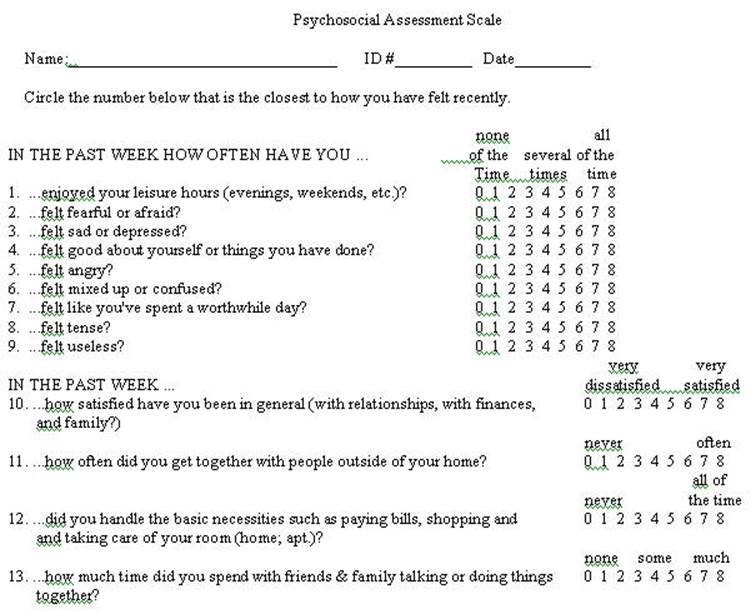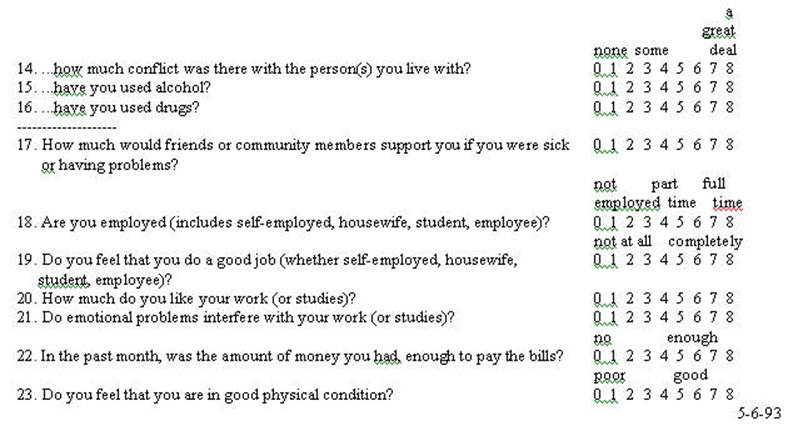The problems throughout this chapter use sample data from the preceding questionnaire. It should be recognized that is selected data -- that is incomplete and selected for the purpose of this example. At the same time is does represent results from a larger study. It is somewhat exaggerated here in that the two samples are: (1) patients at the time of admission to an inpatient hospital and (2) professional staff members. The data is randomly selected from those groups excluding subjects who had missing data. Only 20 cases were selected (10 from each group) so that the mathematical calculations can be followed.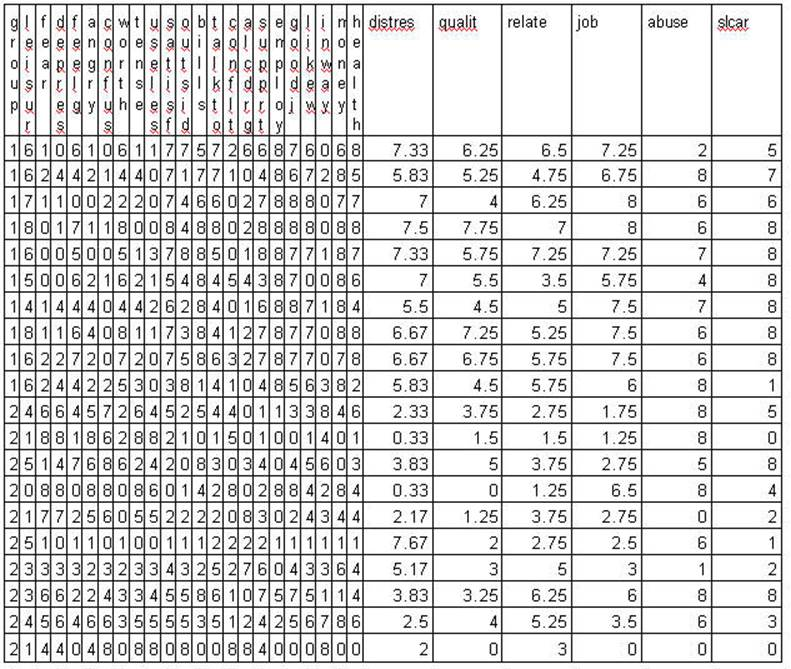A Numerical Example

We are striving to understand the formula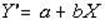most of the general linear model.

The complete General Linear Model also contains an error element

Y'=a + bX + e.

This example deals with only items # 3 and # 8 of the questionnaire. Those questions were "In the past week how often have you felt sad or depressed?" and "In the past week how often have you felt tense?" and are labeled as DEPRES and TENSE respectively. A discussion of the correlation between responses to these two items (items 3 and 8 on the questionnaire) follows.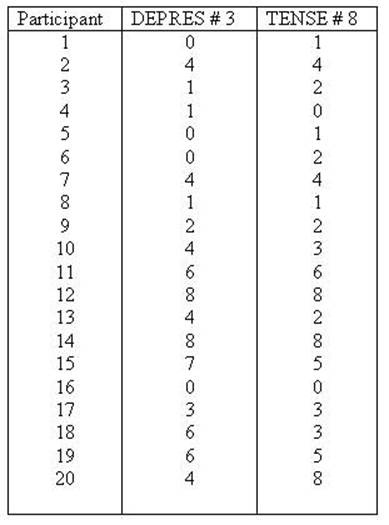We will now present this data in the same way as the more limited data was presented in chapter 2. So that all of the formulae are the same as those presented in chapter 2 you're not learning a new set. This is a more alive example and goes through the same process as in the previous chapter. The variable TENSE is labeled as the X variable (predictor or independent variable) and DEPRES as the Y variable (criterion or dependent variable). First the data will be presented and the SPSS syntax files to compute it will be given.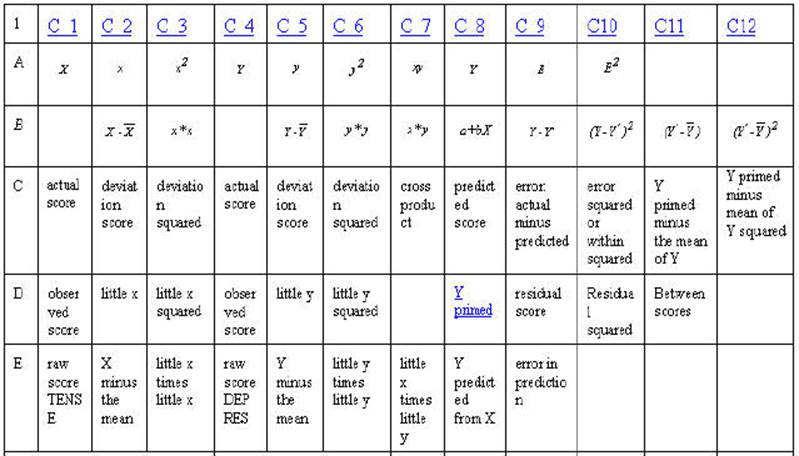Table 2-3. Rows A through F are either mathematical notation or verbal description of mathematical calculations of the numbers in the column. Rows 1 through 20 are associated numbers involved the calculation. Row G is the sum of the numbers in the column while row H is the mean for the column. Row I is the usual verbal description of the sum in the column and row J is an abbreviation of that description.

In the example below when there are scores for all 20 cases are individually computed only the first 4 will be given (this occurs with observation, little x, Y' and SSE).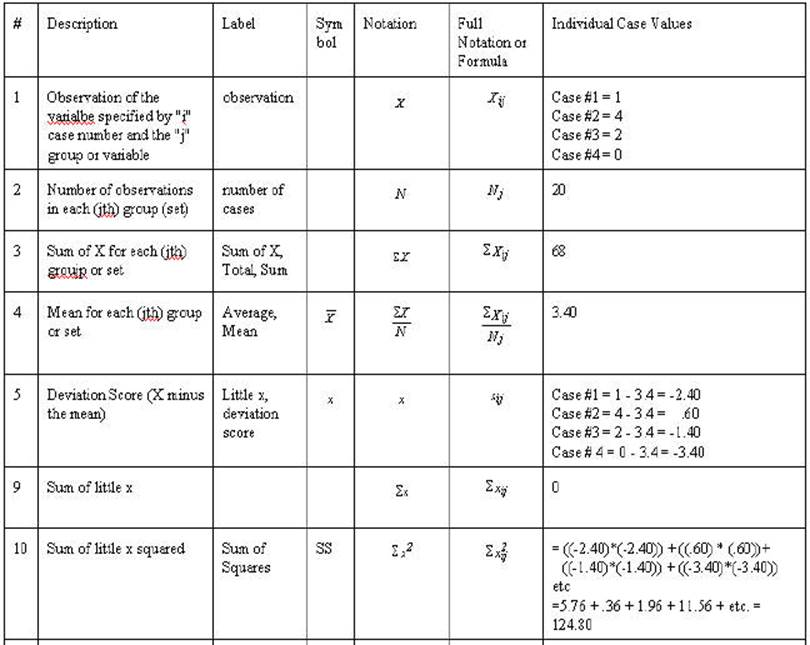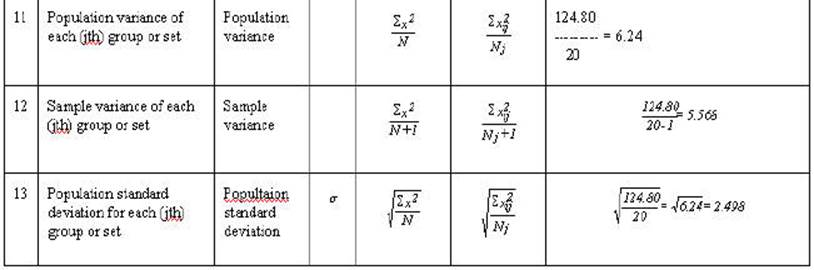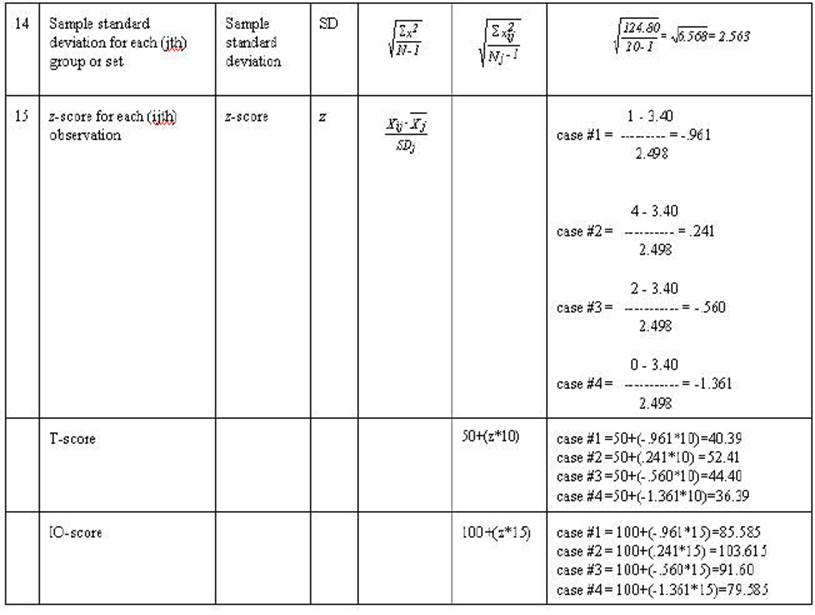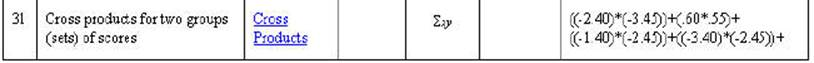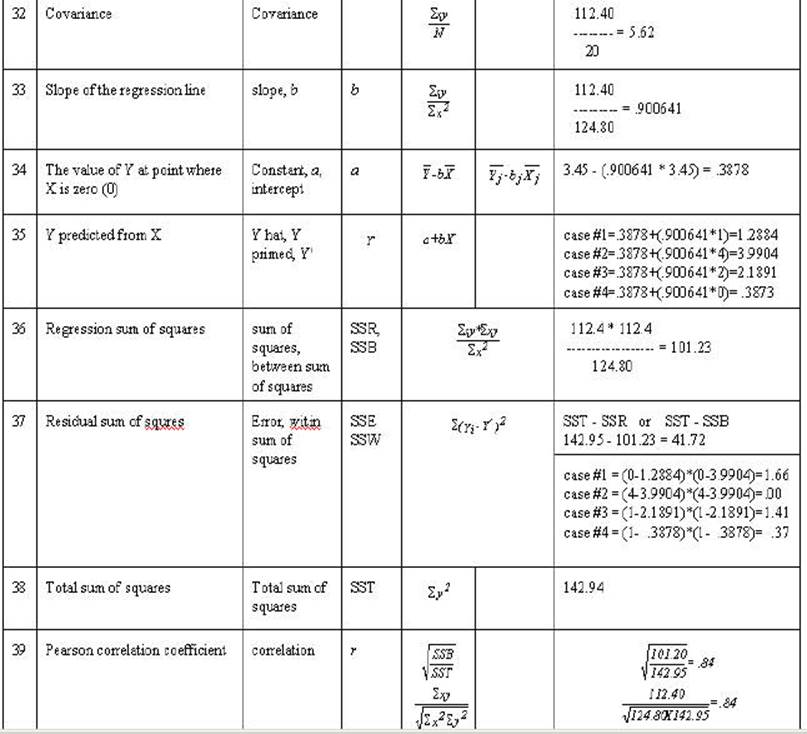The correlation and regression can be shown graphically in terms of the General Linear Model to develop understanding.

Graphic Representation of Sums of Squares RegressionThe correlation and regression can be shown graphically to develop understanding.

This scattergram represents all of the respondents on the items of TENSE and DEPRES. People who responded with smaller numbers to the item TENSE also responded with smaller numbers to DEPRES. At the same time people who responded with larger numbers to TENSE also responded with larger numbers to DEPRES. This plot represents two variables DEPRES and TENSE. Person 16 answered both questions 0. Persons 12 and 14 answered both questions 8. Person 6 responded 2 to TENSE and a 0 to DEPRES. You might want to identify some more of the cases to convince yourself of the relationship of the data to the plot

The next three plots all have the same data as the previous but have modifications drawn to show characteristics of the correlation or regression. The next plot shows the sum of squares due to error or residual. It is the error in predicting Y from X.
TENSE is the X variable and DEPRES is the Y variable.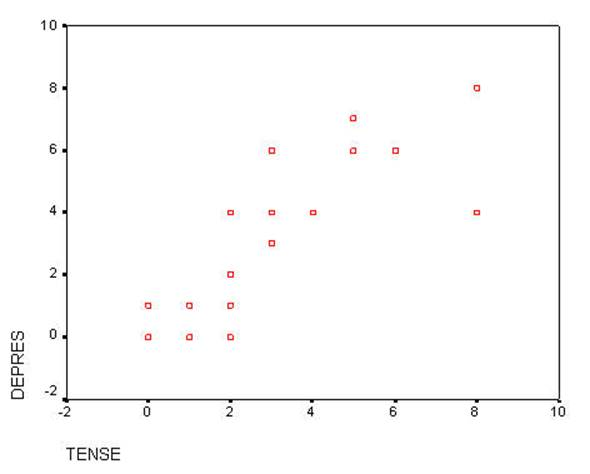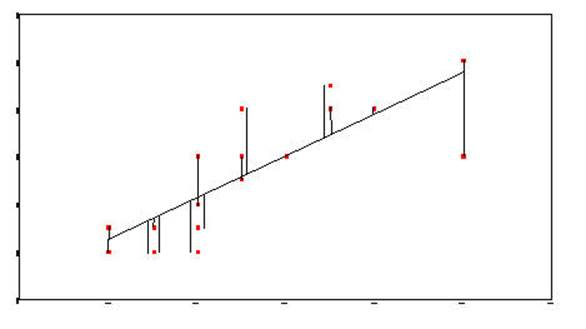Sum-of-Squares-Residual (or Sum-of-Squares-Error) are generated by taking the distance from each data point and the regression line, squaring it, and adding all of the squared distances together.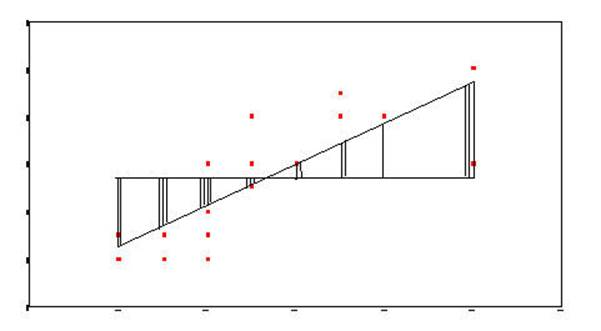Sum-of-Squares-Regression (or Sum-of-Squres-Between) is generated by taking the distance from the mean of Y and the regression line and squaring it.  This is done for each data point.  Each of these squared distances is added together to become the Sum-of-Squares-Regression (Sum-of-Squares-Between).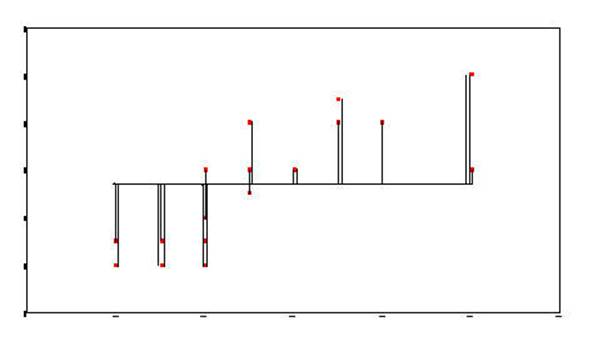The Total-Sum-of-Squares is generated by squaring the distance from the mean of Y and each data point and then summing the squared results.

Graphic Representation of Sums of Squares ANOVA

The t-test can be shown graphically in terms of the General Linear Model to develop understanding.

This plot represents two variables DEPRES and GROUP. There were three people in GROUP # 1 who answered 0 to the question of "sad or depressed." If you look back at the raw data you will that was participants 1, 5, and 6. There was one person in GROUP # 2 that answered the question as 0. In looking at the raw data you will see that it was person # 16. There were two people in GROUP # 2 that answered the question as 8. There were person number # 12 and person # 14. This scattergram represents all the people of both groups. Once again the scattergram represents a relationship. The smaller going with the small and the large with the large. People in GROUP # 1 gave responses which were smaller and people in GROUP # 2 (2 is larger than one) gave responses which were larger than those in GROUP # 1.

The two variables DEPRES and GROUP follow: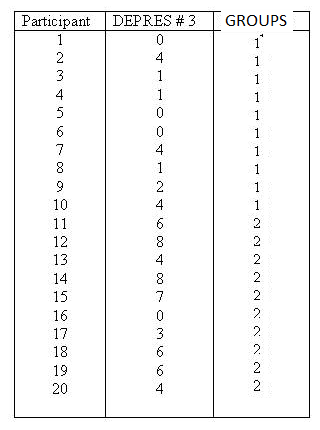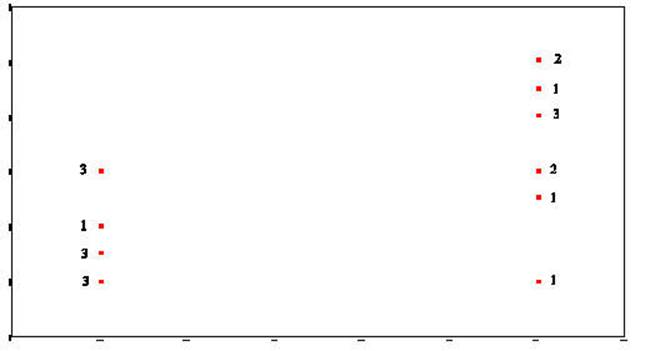Group #1                                                                                                           Group # 2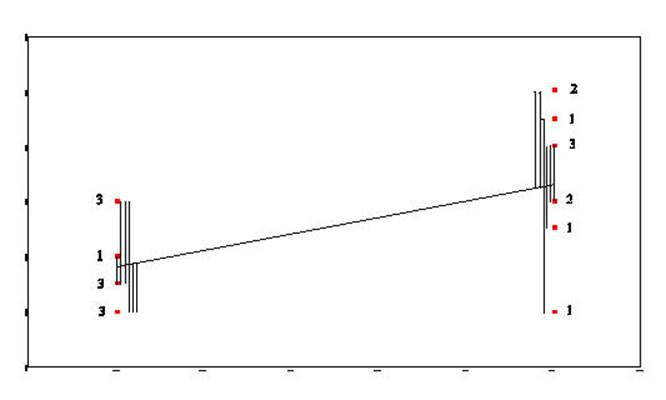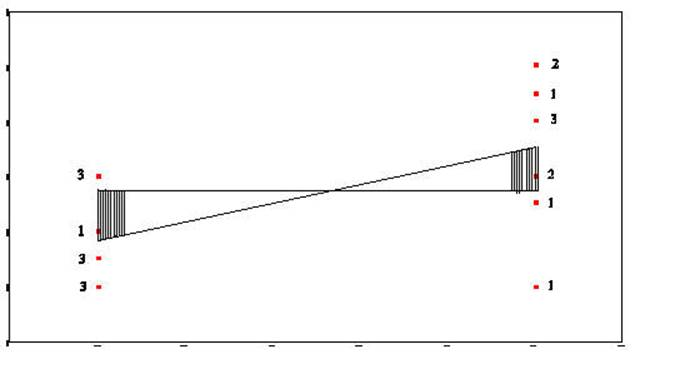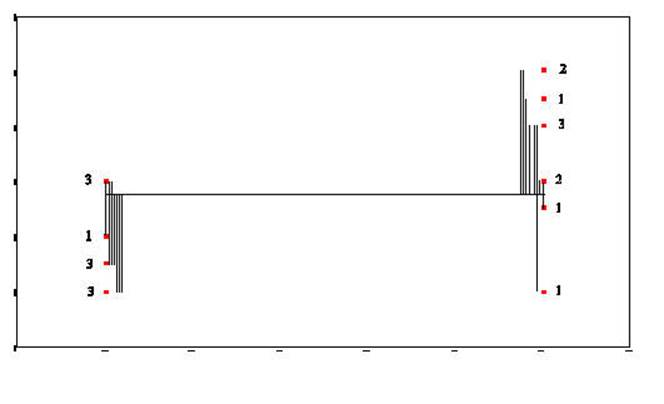Sum-of-Squares-Residual (or Sum-of-Squares-Error) are generated by taking the distance from each data point and the regression line, squaring it, and adding all of the squared distances together.

Sum-of-Squares-Regression (or Sum-of-Squres-Between) is generated by taking the distance from the mean of Y and the regression line and squaring it.  This is done for each data point.  Each of these squared distances is added together to become the Sum-of-Squares-Regression (Sum-of-Squares-Between).

The Total-Sum-of-Squares is generated by squaring the distance from the mean of Y and each data point and then summing the squared results.